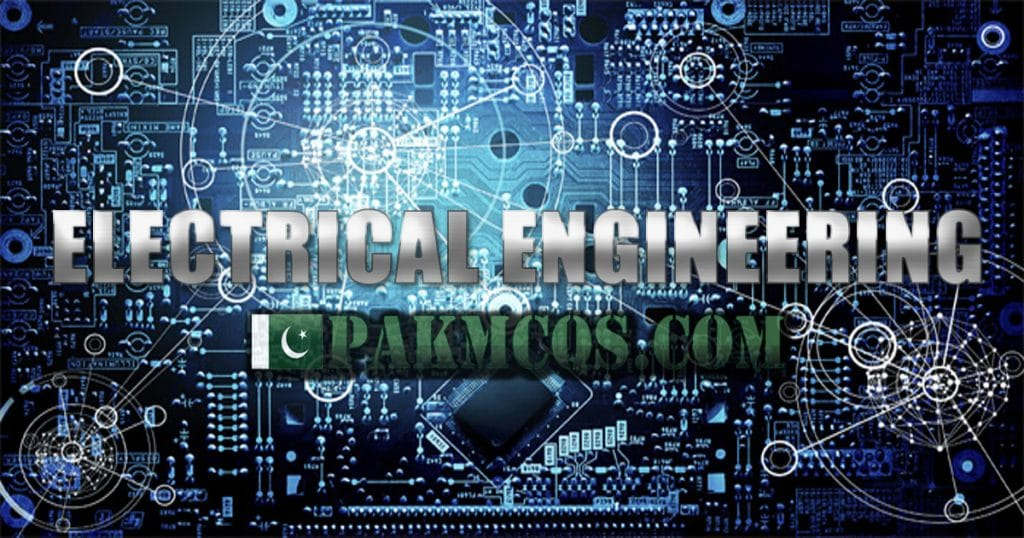A. P
B. 3P
C. P/3
D. None of the above

Assume 3 numbers of pure resistive loads each of value R.

When these loads are connected in delta the power in each resistor is V^2/R and the total power is 3×V^2/R.

When these loads are connected in star the voltage applied across each = V/(sqrt of 3). Power in each load becomes = V^2/(3×R) . and the total load is =(3×V^2)/(3×R)=V^2/R. You can see this is 3 times less than the total power when the loads are connected in delta.

One important point is that the loads should withstand higher voltage (line voltage) and higher current when connected in delta than when they are connected in star
so power in delta is 3 time power correct answer is 3P

Basic Electrical Mcqs
Basic Electrical Mcqs and Quiz with explanation. These objective type Basic Electrical Engineering questions are very important for Job test, semester exams and interviews. Basic Electrical Engineering Mcqs. Basic Concepts (Electrical Fundamentals) MCQs With Explanatory Answers
Electrical Engineering McqsElectrical engineering Mcqs for Preparation of Job Test and interview, freshers, Students, competitive exams etc. Multiple choice questions here are on topics such as Electrical Machines, Power Electronics, Electrical measurement & units, Utilization of Electrical Energy, Basic Electrical Engineering, Electrical Installation, Power Systems, Testing & maintenance of electrical equipment's etc.

ELECTRICAL ENGINEERING MCQS AND QUIZ TEST FOR PREPARATION
ELECTRICAL ENGINEERING MCQS ELECTRICAL ENGINEERING QUIZ
1. Basic Electrical Mcqs 2. Power Electronics Mcqs
3. Transformers 4. Transmission and Distribution
5. D.C. Motors 6. D.C. Generators
7. Polyphase Induction Motors 8. Single Phase Induction Motors
9. Synchronous Motors 10. Network Theorems
11. Economics of Power Generation 12. A.C. Fundamentals, Circuits and Circuit Theory
13. Cables 14. Electrolysis and Storage of Batteries
15. Electrostatics 16. Magnetic Circuit
17. Rectifiers and Converters 18. Magnetism and Electromagnetism
19. Electromagnetic Induction 20. Switchgear and Protection
21. OP-AMP Circuits 22. Heating and Welding
23. Earthing or Grounding 24. Parallel Circuits
25. Oscillators 26. Amplifiers with Negative Feedback
27. TRANSISTORS 28. Semiconductor Diode
29. Analog Electronics 30. Hybrid Parameters
31. Modulation and Demodulation 32. Control Systems
33. Digital Electronics 34. Electric Traction
35. Electrical Engineering Materials 36. Electrical Machine Design
37. Electronic instruments 38. Field Effect Transistors – FET
39. Hybrid Parameters 40. Industrial Drives
41. integrated Circuits 42. Measurement and Instrumentation
43. Multi-Stage Transistor Amplifiers 44. Power Generation
45. Regulated D.C. Power Supply 46. SCR (Silicon Controlled Rectifiers)
47. Semiconductor Theory 48. Single Stage Transistor Amplifiers
49. Solid-State Switching Circuits 50. Transistor Audio Power Amplifiers
51. Transistor Biasing 52. Transistor Tuned Amplifiers
53. Series-parallel Circuits 54. Passive Filters

IF YOU THINK THAT ABOVE POSTED MCQ IS WRONG.

###### 3 Comments to “ If P is the power of a star connected system then what will be power of an equivalent delta connected system?”
1. abid says:

welldone.. Very good question